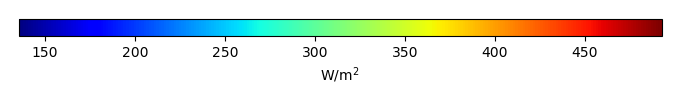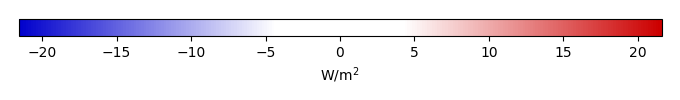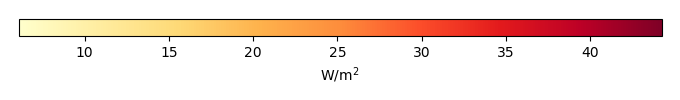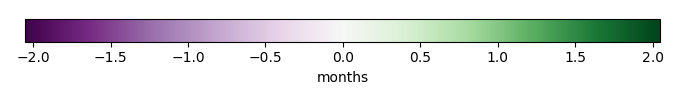# Mean State

Period Mean (original grids) [W/m2]
Model Period Mean (intersection) [W/m2]
Model Period Mean (complement) [W/m2]
Benchmark Period Mean (intersection) [W/m2]
Benchmark Period Mean (complement) [W/m2]
Bias [W/m2]
RMSE [W/m2]
Phase Shift [months]
Bias Score 
RMSE Score 
Seasonal Cycle Score 
Spatial Distribution Score 
Interannual Variability Score 
Overall Score 
Benchmark [-] 457.
CLM5PHSOFF [-] 464. 464. 456. 465. 7.94 16.8 1.52 0.433 0.377 0.789 0.972 0.626 0.596
CLM5PHSON [-] 463. 463. 456. 465. 7.83 16.4 1.44 0.460 0.382 0.805 0.979 0.612 0.603
Period Mean (original grids) [W/m2]
Model Period Mean (intersection) [W/m2]
Model Period Mean (complement) [W/m2]
Benchmark Period Mean (intersection) [W/m2]
Benchmark Period Mean (complement) [W/m2]
Bias [W/m2]
RMSE [W/m2]
Phase Shift [months]
Bias Score 
RMSE Score 
Seasonal Cycle Score 
Spatial Distribution Score 
Interannual Variability Score 
Overall Score 
Benchmark [-] 466.
CLM5PHSOFF [-] 484. 484. 467. 458. 17.4 23.4 0.546 0.455 0.511 0.953 0.915 0.647 0.665
CLM5PHSON [-] 484. 484. 467. 458. 17.2 23.5 0.637 0.460 0.502 0.942 0.951 0.622 0.663
Period Mean (original grids) [W/m2]
Model Period Mean (intersection) [W/m2]
Model Period Mean (complement) [W/m2]
Benchmark Period Mean (intersection) [W/m2]
Benchmark Period Mean (complement) [W/m2]
Bias [W/m2]
RMSE [W/m2]
Phase Shift [months]
Bias Score 
RMSE Score 
Seasonal Cycle Score 
Spatial Distribution Score 
Interannual Variability Score 
Overall Score 
Benchmark [-] 443.
CLM5PHSOFF [-] 454. 454. 443. 446. 11.6 18.9 1.04 0.520 0.498 0.860 0.999 0.641 0.669
CLM5PHSON [-] 452. 452. 443. 446. 9.13 18.8 1.38 0.570 0.463 0.814 1.00 0.603 0.652
Period Mean (original grids) [W/m2]
Model Period Mean (intersection) [W/m2]
Model Period Mean (complement) [W/m2]
Benchmark Period Mean (intersection) [W/m2]
Benchmark Period Mean (complement) [W/m2]
Bias [W/m2]
RMSE [W/m2]
Phase Shift [months]
Bias Score 
RMSE Score 
Seasonal Cycle Score 
Spatial Distribution Score 
Interannual Variability Score 
Overall Score 
Benchmark [-] 279.
CLM5PHSOFF [-] 280. 280. 278. 296. 2.48 15.5 0.126 0.900 0.809 0.990 1.00 0.746 0.876
CLM5PHSON [-] 277. 278. 278. 296. 2.37 15.4 0.127 0.903 0.808 0.990 1.00 0.744 0.875
Period Mean (original grids) [W/m2]
Model Period Mean (intersection) [W/m2]
Model Period Mean (complement) [W/m2]
Benchmark Period Mean (intersection) [W/m2]
Benchmark Period Mean (complement) [W/m2]
Bias [W/m2]
RMSE [W/m2]
Phase Shift [months]
Bias Score 
RMSE Score 
Seasonal Cycle Score 
Spatial Distribution Score 
Interannual Variability Score 
Overall Score 
Benchmark [-] 457.
CLM5PHSOFF [-] 459. 459. 454. 467. 4.79 14.7 1.53 0.285 0.376 0.767 0.659 0.585 0.508
CLM5PHSON [-] 459. 459. 454. 467. 5.54 14.6 1.55 0.286 0.378 0.766 0.648 0.579 0.506
Period Mean (original grids) [W/m2]
Model Period Mean (intersection) [W/m2]
Model Period Mean (complement) [W/m2]
Benchmark Period Mean (intersection) [W/m2]
Benchmark Period Mean (complement) [W/m2]
Bias [W/m2]
RMSE [W/m2]
Phase Shift [months]
Bias Score 
RMSE Score 
Seasonal Cycle Score 
Spatial Distribution Score 
Interannual Variability Score 
Overall Score 
Benchmark [-] 312.
CLM5PHSOFF [-] 318. 318. 311. 334. 6.89 12.9 0.0457 0.894 0.877 0.997 1.00 0.865 0.918
CLM5PHSON [-] 317. 317. 311. 334. 5.51 11.6 0.0521 0.909 0.884 0.997 0.999 0.859 0.922
Period Mean (original grids) [W/m2]
Model Period Mean (intersection) [W/m2]
Model Period Mean (complement) [W/m2]
Benchmark Period Mean (intersection) [W/m2]
Benchmark Period Mean (complement) [W/m2]
Bias [W/m2]
RMSE [W/m2]
Phase Shift [months]
Bias Score 
RMSE Score 
Seasonal Cycle Score 
Spatial Distribution Score 
Interannual Variability Score 
Overall Score 
Benchmark [-] 364.
CLM5PHSOFF [-] 369. 368. 363. 399. 5.34 14.6 0.138 0.848 0.825 0.990 0.997 0.760 0.874
CLM5PHSON [-] 366. 365. 363. 399. 4.07 14.2 0.164 0.858 0.822 0.988 0.996 0.762 0.875
Period Mean (original grids) [W/m2]
Model Period Mean (intersection) [W/m2]
Model Period Mean (complement) [W/m2]
Benchmark Period Mean (intersection) [W/m2]
Benchmark Period Mean (complement) [W/m2]
Bias [W/m2]
RMSE [W/m2]
Phase Shift [months]
Bias Score 
RMSE Score 
Seasonal Cycle Score 
Spatial Distribution Score 
Interannual Variability Score 
Overall Score 
Benchmark [-] 448.
CLM5PHSOFF [-] 457. 458. 446. 467. 11.6 19.4 0.677 0.569 0.574 0.932 0.981 0.641 0.712
CLM5PHSON [-] 453. 454. 446. 467. 9.50 18.9 0.825 0.605 0.557 0.913 0.979 0.628 0.707
Period Mean (original grids) [W/m2]
Model Period Mean (intersection) [W/m2]
Model Period Mean (complement) [W/m2]
Benchmark Period Mean (intersection) [W/m2]
Benchmark Period Mean (complement) [W/m2]
Bias [W/m2]
RMSE [W/m2]
Phase Shift [months]
Bias Score 
RMSE Score 
Seasonal Cycle Score 
Spatial Distribution Score 
Interannual Variability Score 
Overall Score 
Benchmark [-] 281.
CLM5PHSOFF [-] 285. 285. 281. 288. 4.57 13.4 0.0859 0.910 0.827 0.993 0.999 0.764 0.887
CLM5PHSON [-] 284. 284. 281. 288. 4.17 13.2 0.0840 0.914 0.827 0.993 1.00 0.767 0.888
Period Mean (original grids) [W/m2]
Model Period Mean (intersection) [W/m2]
Model Period Mean (complement) [W/m2]
Benchmark Period Mean (intersection) [W/m2]
Benchmark Period Mean (complement) [W/m2]
Bias [W/m2]
RMSE [W/m2]
Phase Shift [months]
Bias Score 
RMSE Score 
Seasonal Cycle Score 
Spatial Distribution Score 
Interannual Variability Score 
Overall Score 
Benchmark [-] 441.
CLM5PHSOFF [-] 447. 447. 443. 426. 4.58 13.1 0.346 0.787 0.699 0.970 0.993 0.756 0.817
CLM5PHSON [-] 449. 449. 443. 426. 3.62 13.2 0.381 0.797 0.692 0.969 0.990 0.739 0.813
Period Mean (original grids) [W/m2]
Model Period Mean (intersection) [W/m2]
Model Period Mean (complement) [W/m2]
Benchmark Period Mean (intersection) [W/m2]
Benchmark Period Mean (complement) [W/m2]
Bias [W/m2]
RMSE [W/m2]
Phase Shift [months]
Bias Score 
RMSE Score 
Seasonal Cycle Score 
Spatial Distribution Score 
Interannual Variability Score 
Overall Score 
Benchmark [-] 368.
CLM5PHSOFF [-] 369. 369. 366. 389. 3.05 12.2 0.326 0.847 0.774 0.978 1.00 0.783 0.859
CLM5PHSON [-] 365. 365. 366. 389. 1.41 11.8 0.429 0.859 0.773 0.971 0.999 0.781 0.859
Period Mean (original grids) [W/m2]
Model Period Mean (intersection) [W/m2]
Model Period Mean (complement) [W/m2]
Benchmark Period Mean (intersection) [W/m2]
Benchmark Period Mean (complement) [W/m2]
Bias [W/m2]
RMSE [W/m2]
Phase Shift [months]
Bias Score 
RMSE Score 
Seasonal Cycle Score 
Spatial Distribution Score 
Interannual Variability Score 
Overall Score 
Benchmark [-] 399.
CLM5PHSOFF [-] 384. 384. 376. 409. 7.31 16.5 0.495 0.708 0.669 0.944 1.00 0.700 0.782
CLM5PHSON [-] 381. 381. 376. 409. 6.29 16.1 0.539 0.722 0.667 0.940 0.999 0.692 0.781
Period Mean (original grids) [W/m2]
Model Period Mean (intersection) [W/m2]
Model Period Mean (complement) [W/m2]
Benchmark Period Mean (intersection) [W/m2]
Benchmark Period Mean (complement) [W/m2]
Bias [W/m2]
RMSE [W/m2]
Phase Shift [months]
Bias Score 
RMSE Score 
Seasonal Cycle Score 
Spatial Distribution Score 
Interannual Variability Score 
Overall Score 
Benchmark [-] 450.
CLM5PHSOFF [-] 453. 453. 450. 446. 2.85 15.2 0.338 0.842 0.760 0.975 0.998 0.715 0.842
CLM5PHSON [-] 452. 452. 450. 446. 2.24 15.1 0.358 0.844 0.760 0.973 1.00 0.716 0.842
Period Mean (original grids) [W/m2]
Model Period Mean (intersection) [W/m2]
Model Period Mean (complement) [W/m2]
Benchmark Period Mean (intersection) [W/m2]
Benchmark Period Mean (complement) [W/m2]
Bias [W/m2]
RMSE [W/m2]
Phase Shift [months]
Bias Score 
RMSE Score 
Seasonal Cycle Score 
Spatial Distribution Score 
Interannual Variability Score 
Overall Score 
Benchmark [-] 281.
CLM5PHSOFF [-] 287. 287. 281. 293. 6.39 16.7 0.173 0.884 0.793 0.986 1.00 0.762 0.870
CLM5PHSON [-] 287. 286. 281. 293. 6.06 16.8 0.168 0.888 0.790 0.987 1.00 0.763 0.870
Period Mean (original grids) [W/m2]
Model Period Mean (intersection) [W/m2]
Model Period Mean (complement) [W/m2]
Benchmark Period Mean (intersection) [W/m2]
Benchmark Period Mean (complement) [W/m2]
Bias [W/m2]
RMSE [W/m2]
Phase Shift [months]
Bias Score 
RMSE Score 
Seasonal Cycle Score 
Spatial Distribution Score 
Interannual Variability Score 
Overall Score 
Benchmark [-] 442.
CLM5PHSOFF [-] 453. 452. 439. 459. 13.7 19.7 0.842 0.482 0.558 0.893 0.946 0.688 0.687
CLM5PHSON [-] 447. 447. 439. 459. 12.0 18.6 0.885 0.510 0.561 0.893 0.950 0.672 0.691
Period Mean (original grids) [W/m2]
Model Period Mean (intersection) [W/m2]
Model Period Mean (complement) [W/m2]
Benchmark Period Mean (intersection) [W/m2]
Benchmark Period Mean (complement) [W/m2]
Bias [W/m2]
RMSE [W/m2]
Phase Shift [months]
Bias Score 
RMSE Score 
Seasonal Cycle Score 
Spatial Distribution Score 
Interannual Variability Score 
Overall Score 
Benchmark [-] 386.
CLM5PHSOFF [-] 396. 396. 384. 429. 11.4 15.0 0.102 0.783 0.857 0.993 0.994 0.859 0.890
CLM5PHSON [-] 391. 391. 384. 429. 8.89 13.0 0.112 0.823 0.856 0.992 0.993 0.853 0.896
Period Mean (original grids) [W/m2]
Model Period Mean (intersection) [W/m2]
Model Period Mean (complement) [W/m2]
Benchmark Period Mean (intersection) [W/m2]
Benchmark Period Mean (complement) [W/m2]
Bias [W/m2]
RMSE [W/m2]
Phase Shift [months]
Bias Score 
RMSE Score 
Seasonal Cycle Score 
Spatial Distribution Score 
Interannual Variability Score 
Overall Score 
Benchmark [-] 324.
CLM5PHSOFF [-] 331. 331. 323. 347. 7.47 14.1 0.0627 0.861 0.832 0.995 0.984 0.787 0.882
CLM5PHSON [-] 328. 328. 323. 347. 5.87 13.2 0.104 0.881 0.831 0.993 0.972 0.781 0.882
Period Mean (original grids) [W/m2]
Model Period Mean (intersection) [W/m2]
Model Period Mean (complement) [W/m2]
Benchmark Period Mean (intersection) [W/m2]
Benchmark Period Mean (complement) [W/m2]
Bias [W/m2]
RMSE [W/m2]
Phase Shift [months]
Bias Score 
RMSE Score 
Seasonal Cycle Score 
Spatial Distribution Score 
Interannual Variability Score 
Overall Score 
Benchmark [-] 377.
CLM5PHSOFF [-] 383. 384. 377. 378. 7.04 14.9 0.0975 0.827 0.811 0.993 1.00 0.747 0.865
CLM5PHSON [-] 381. 382. 377. 378. 5.35 14.1 0.110 0.842 0.814 0.992 1.00 0.743 0.867
Period Mean (original grids) [W/m2]
Model Period Mean (intersection) [W/m2]
Model Period Mean (complement) [W/m2]
Benchmark Period Mean (intersection) [W/m2]
Benchmark Period Mean (complement) [W/m2]
Bias [W/m2]
RMSE [W/m2]
Phase Shift [months]
Bias Score 
RMSE Score 
Seasonal Cycle Score 
Spatial Distribution Score 
Interannual Variability Score 
Overall Score 
Benchmark [-] 449.
CLM5PHSOFF [-] 459. 459. 449. 463. 9.62 15.3 1.23 0.318 0.391 0.836 0.972 0.643 0.592
CLM5PHSON [-] 458. 458. 449. 463. 9.09 14.8 1.21 0.318 0.399 0.836 0.991 0.625 0.595
Period Mean (original grids) [W/m2]
Model Period Mean (intersection) [W/m2]
Model Period Mean (complement) [W/m2]
Benchmark Period Mean (intersection) [W/m2]
Benchmark Period Mean (complement) [W/m2]
Bias [W/m2]
RMSE [W/m2]
Phase Shift [months]
Bias Score 
RMSE Score 
Seasonal Cycle Score 
Spatial Distribution Score 
Interannual Variability Score 
Overall Score 
Benchmark [-] 443.
CLM5PHSOFF [-] 451. 451. 443. 434. 7.56 14.8 0.773 0.652 0.571 0.889 0.994 0.689 0.728
CLM5PHSON [-] 449. 449. 443. 434. 5.44 14.2 0.895 0.695 0.564 0.868 0.992 0.663 0.724
Period Mean (original grids) [W/m2]
Model Period Mean (intersection) [W/m2]
Model Period Mean (complement) [W/m2]
Benchmark Period Mean (intersection) [W/m2]
Benchmark Period Mean (complement) [W/m2]
Bias [W/m2]
RMSE [W/m2]
Phase Shift [months]
Bias Score 
RMSE Score 
Seasonal Cycle Score 
Spatial Distribution Score 
Interannual Variability Score 
Overall Score 
Benchmark [-] 383.
CLM5PHSOFF [-] 390. 389. 381. 396. 7.35 21.0 0.924 0.610 0.566 0.884 0.999 0.629 0.709
CLM5PHSON [-] 387. 386. 381. 396. 5.53 20.2 0.891 0.632 0.570 0.890 0.998 0.636 0.716

# Temporally integrated period mean

BENCHMARK MEANMODEL MEANBIASBIAS SCORERMSERMSE SCOREBENCHMARK INTERANNUAL VARIABILITYMODEL INTERANNUAL VARIABILITYINTERANNUAL VARIABILITY SCOREBENCHMARK MAX MONTHMODEL MAX MONTHDIFFERENCE IN MAX MONTHSEASONAL CYCLE SCORESPATIAL TAYLOR DIAGRAMMODEL COLORS# Spatially integrated regional mean

MODEL COLORSREGIONAL MEANANNUAL CYCLEMONTHLY ANOMALYANNUAL CYCLE# All Models

BenchmarkCLM5PHSOFFCLM5PHSON# Data Information

creation_date: Thu May 8 23:13:11 PDT 2014

source_file: This product is generated from monthly 1 degree CERES EBAF Radiation observations